# RS Aggarwal Solutions for Class 8 Maths Chapter 20 - Volume and Surface Area of Solids Exercise 20C

RS Aggarwal Solutions for the Exercise 20C of Class 8 Maths Chapter 20, Volume and Surface Area of Solids are available here. BYJU’S expert has uniquely solved the exercise. As this is the last exercise, it includes multiple-choice questions which enhances time management among the students. Practising as many times as possible helps in developing time management skills and also boosts the confidence level to achieve high marks. By practising the RS Aggarwal Solutions for Class 8, students will be able to grasp the concepts correctly.

## Download PDF of RS Aggarwal Solutions for Class 8 Maths Chapter 20 – Volume And Surface Area Of Solids – Exercise 20C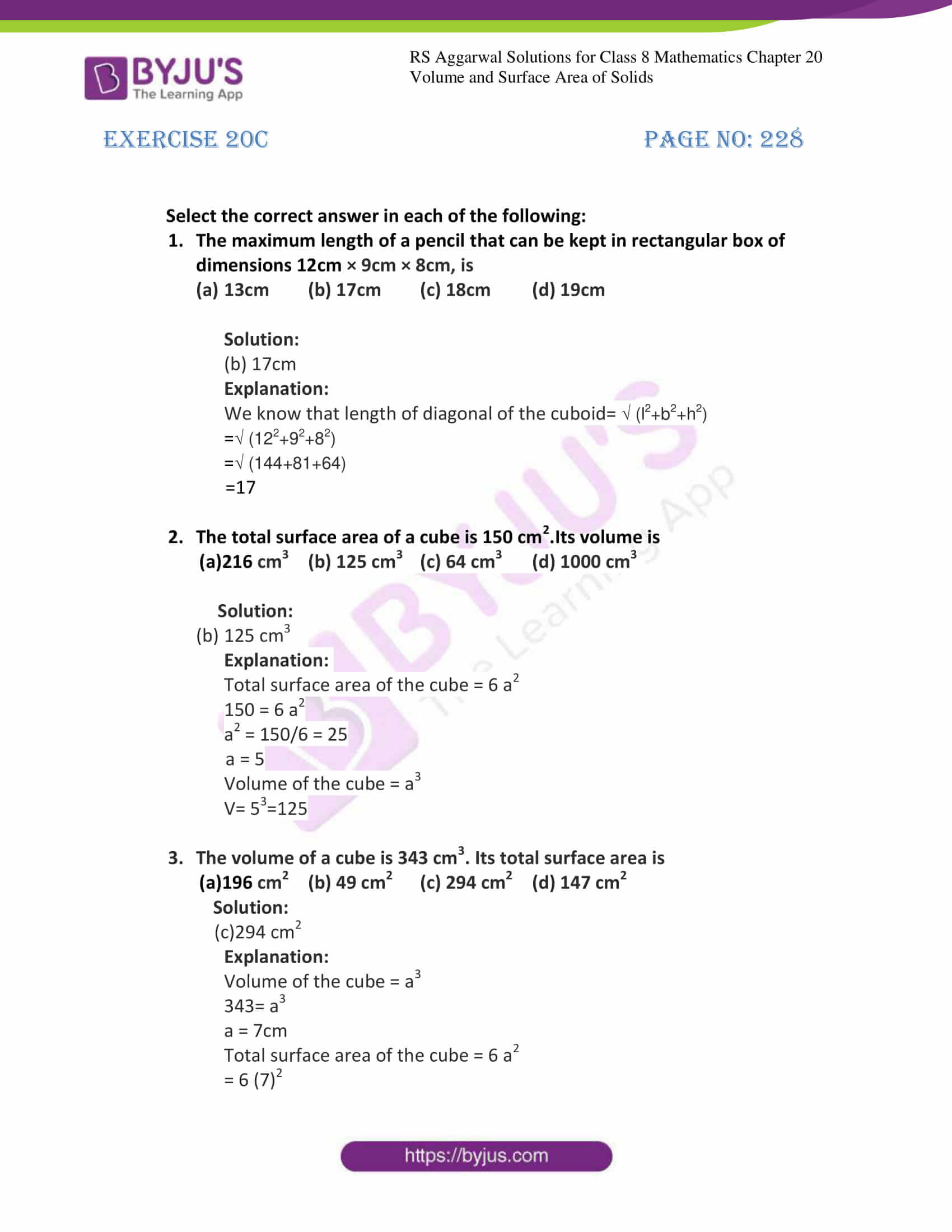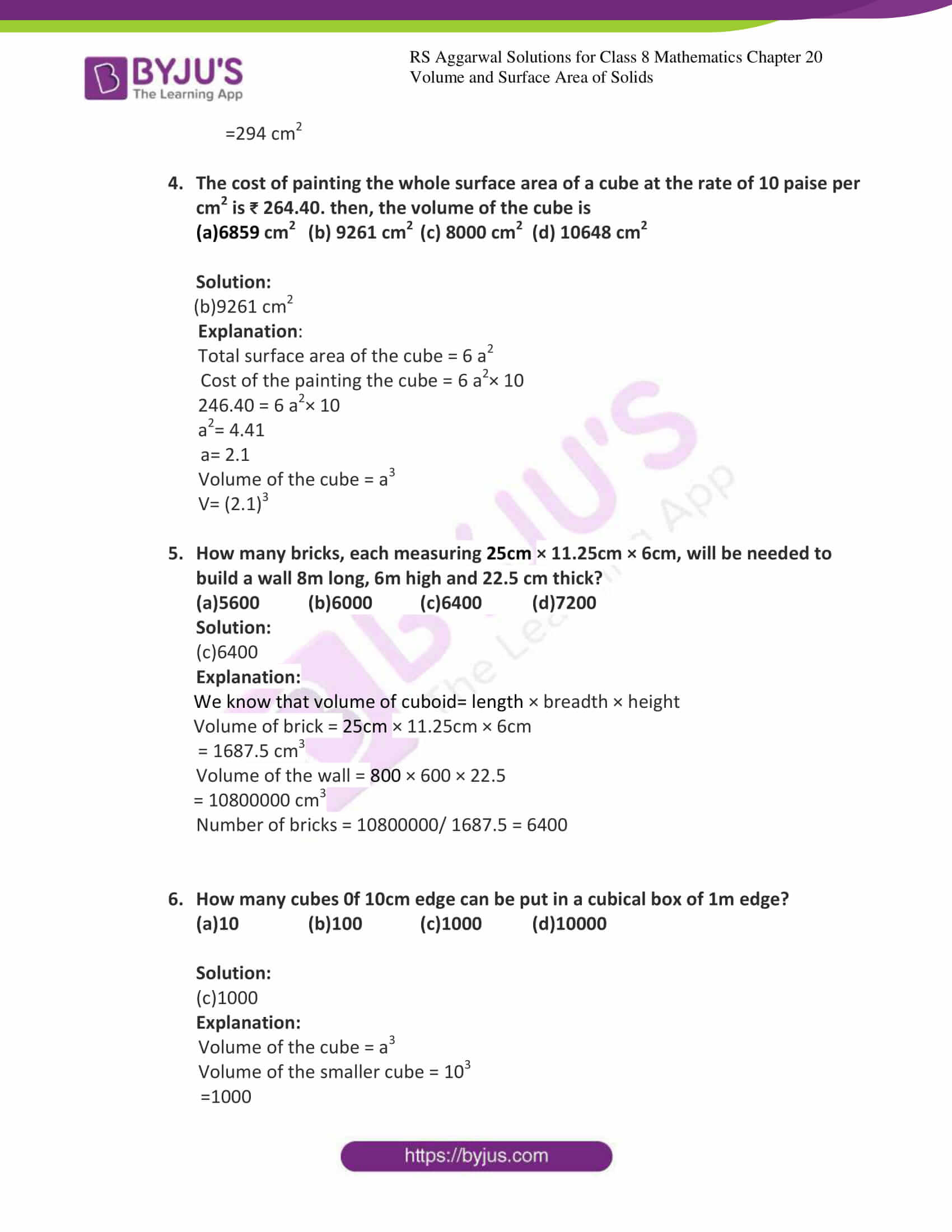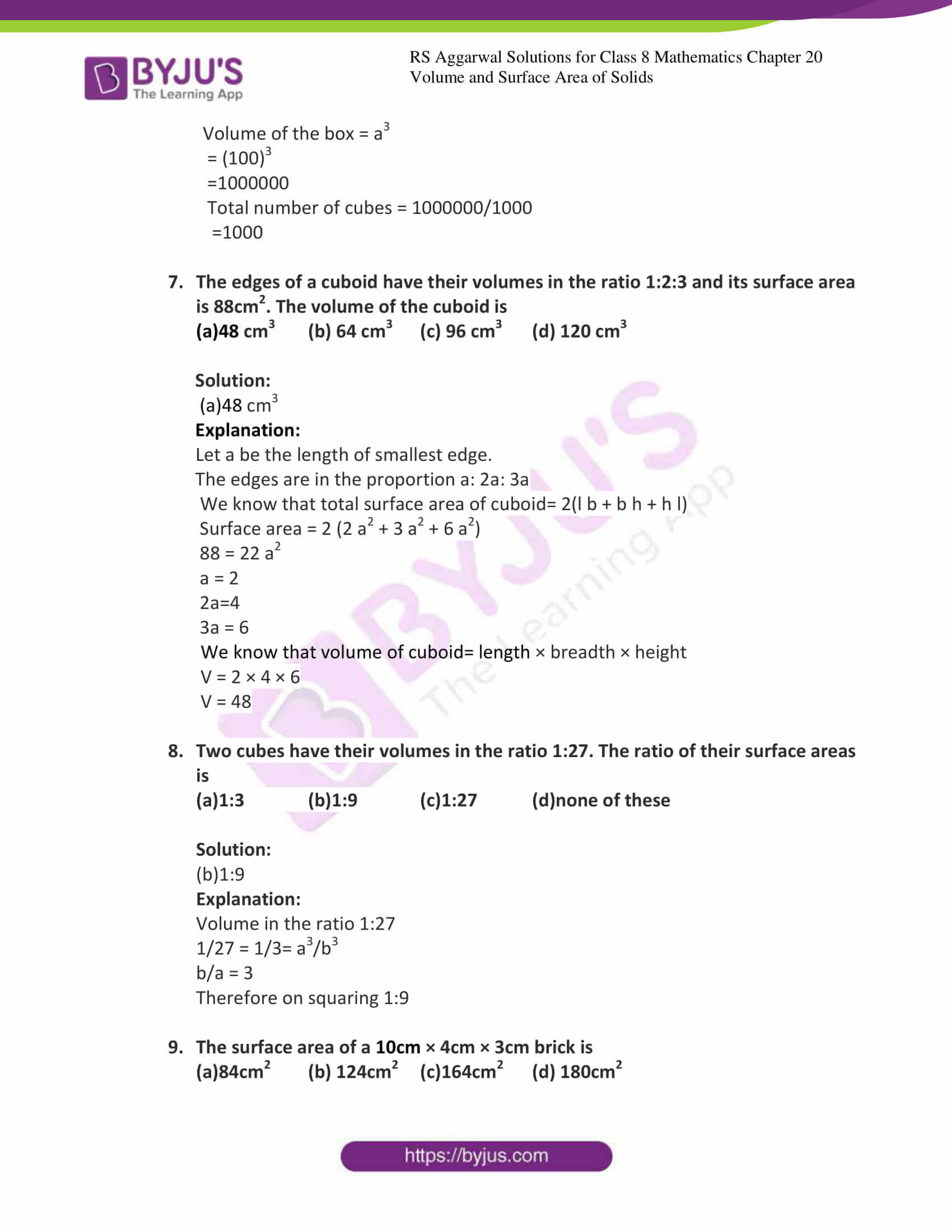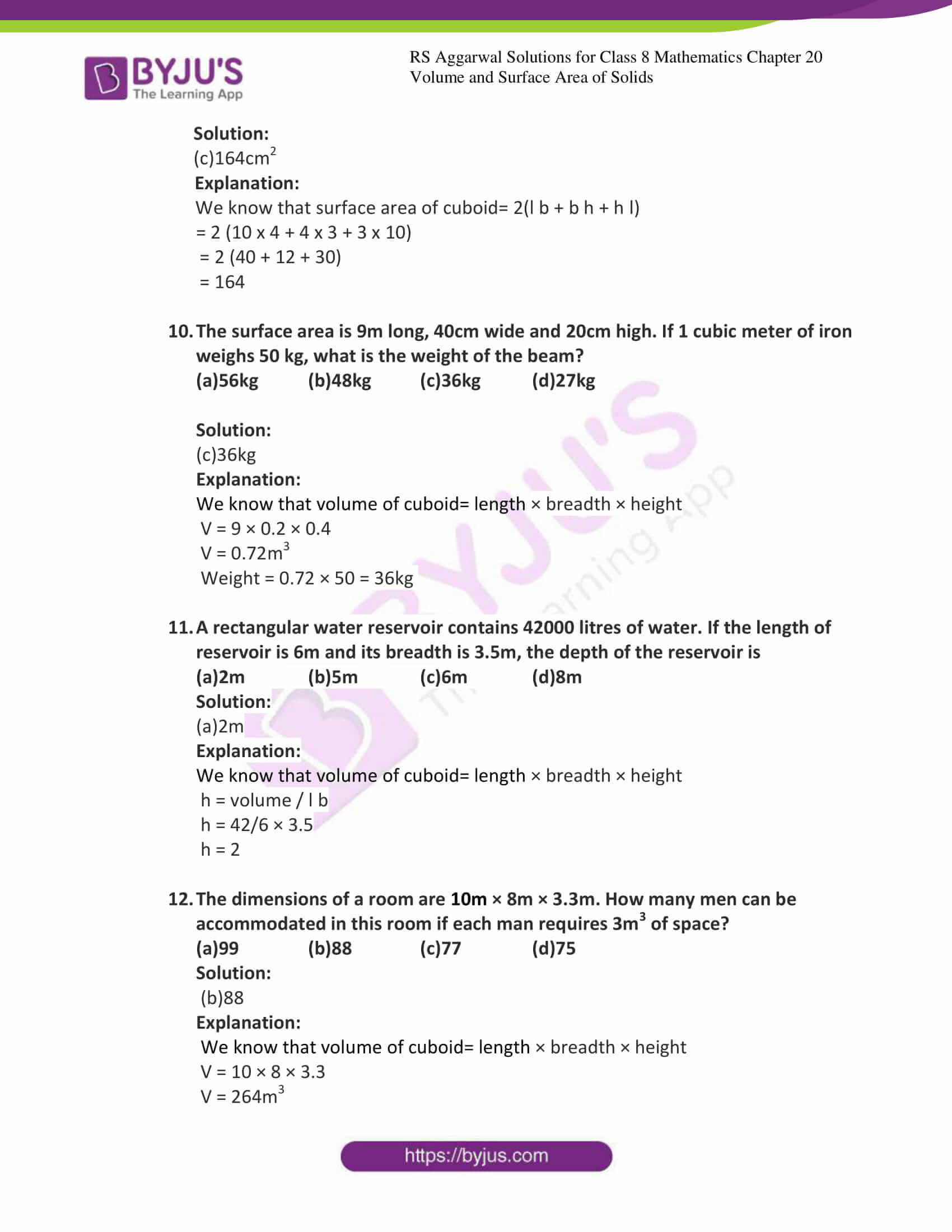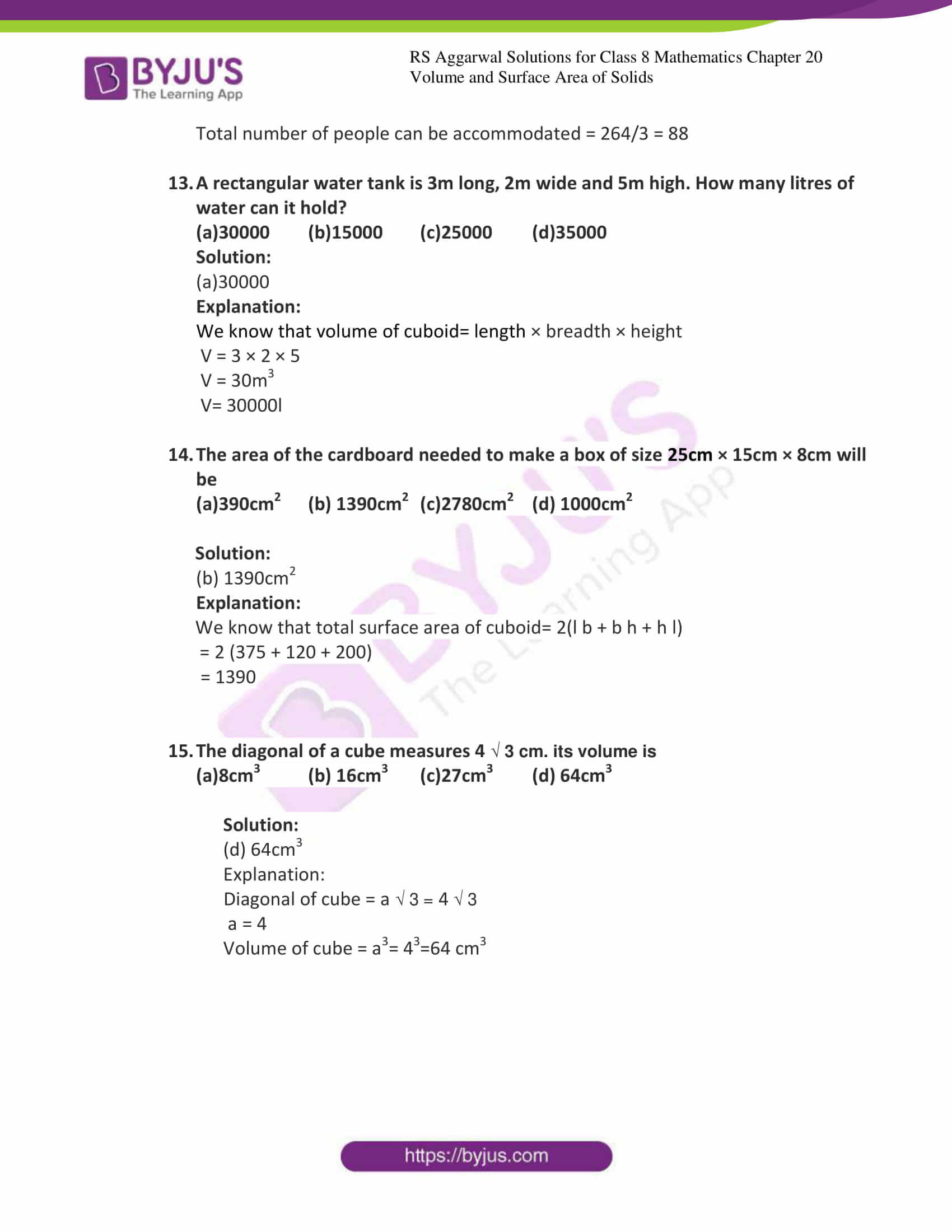### Access answers to Maths RS Aggarwal Solutions for Class 8 Chapter 20 – Volume and Surface Area of Solids Exercise 20C

Exercise 20C Page No: 228

Select the correct answer in each of the following:

1. The maximum length of a pencil that can be kept in rectangular box of dimensions 12cm × 9cm × 8cm, is

(a) 13cm (b) 17cm (c) 18cm (d) 19cm

Solution:

(b) 17cm

Explanation:

We know that length of diagonal of the cuboid= √ (l2+b2+h2)

=√ (122+92+82)

=√ (144+81+64)

=17

2. The total surface area of a cube is 150 cm2.Its volume is

(a)216 cm3 (b) 125 cm3 (c) 64 cm3 (d) 1000 cm3

Solution:

1. 125 cm3

Explanation:

Total surface area of the cube = 6 a2

150 = 6 a2

a2 = 150/6 = 25

a = 5

Volume of the cube = a3

V= 53=125

3. The volume of a cube is 343 cm3. Its total surface area is

(a)196 cm2 (b) 49 cm2 (c) 294 cm2 (d) 147 cm2

Solution:

Explanation:

Volume of the cube = a3

343= a3

a = 7cm

Total surface area of the cube = 6 a2

= 6 (7)2

=294 cm2

4. The cost of painting the whole surface area of a cube at the rate of 10 paise per cm2 is ₹ 264.40. then, the volume of the cube is

(a)6859 cm2 (b) 9261 cm2 (c) 8000 cm2 (d) 10648 cm2

Solution:

(b)9261 cm2

Explanation:

Total surface area of the cube = 6 a2

Cost of the painting the cube = 6 a2× 10

246.40 = 6 a2× 10

a2= 4.41

a= 2.1

Volume of the cube = a3

V= (2.1)3

5. How many bricks, each measuring 25cm × 11.25cm × 6cm, will be needed to build a wall 8m long, 6m high and 22.5 cm thick?

(a)5600 (b)6000 (c)6400 (d)7200

Solution:

Explanation:

We know that volume of cuboid= length × breadth × height

Volume of brick = 25cm × 11.25cm × 6cm

= 1687.5 cm3

Volume of the wall = 800 × 600 × 22.5

= 10800000 cm3

Number of bricks = 10800000/ 1687.5 = 6400

6. How many cubes 0f 10cm edge can be put in a cubical box of 1m edge?

(a)10 (b)100 (c)1000 (d)10000

Solution:

Explanation:

Volume of the cube = a3

Volume of the smaller cube = 103

=1000

Volume of the box = a3

= (100)3

=1000000

Total number of cubes = 1000000/1000

=1000

7. The edges of a cuboid have their volumes in the ratio 1:2:3 and its surface area is 88cm2. The volume of the cuboid is

(a)48 cm3 (b) 64 cm3 (c) 96 cm3 (d) 120 cm3

Solution:

(a)48 cm3

Explanation:

Let a be the length of smallest edge.

The edges are in the proportion a: 2a: 3a

We know that total surface area of cuboid= 2(l b + b h + h l)

Surface area = 2 (2 a2 + 3 a2 + 6 a2)

88 = 22 a2

a = 2

2a=4

3a = 6

We know that volume of cuboid= length × breadth × height

V = 2 × 4 × 6

V = 48

8. Two cubes have their volumes in the ratio 1:27. The ratio of their surface areas is

(a)1:3 (b)1:9 (c)1:27 (d)none of these

Solution:

(b)1:9

Explanation:

Volume in the ratio 1:27

1/27 = 1/3= a3/b3

b/a = 3

Therefore on squaring 1:9

9. The surface area of a 10cm × 4cm × 3cm brick is

(a)84cm2 (b) 124cm2 (c)164cm2 (d) 180cm2

Solution:

Explanation:

We know that surface area of cuboid= 2(l b + b h + h l)

= 2 (10 x 4 + 4 x 3 + 3 x 10)

= 2 (40 + 12 + 30)

= 164

10. The surface area is 9m long, 40cm wide and 20cm high. If 1 cubic meter of iron weighs 50 kg, what is the weight of the beam?

(a)56kg (b)48kg (c)36kg (d)27kg

Solution:

Explanation:

We know that volume of cuboid= length × breadth × height

V = 9 × 0.2 × 0.4

V = 0.72m3

Weight = 0.72 × 50 = 36kg

11. A rectangular water reservoir contains 42000 litres of water. If the length of reservoir is 6m and its breadth is 3.5m, the depth of the reservoir is

(a)2m (b)5m (c)6m (d)8m

Solution:

(a)2m

Explanation:

We know that volume of cuboid= length × breadth × height

h = volume / l b

h = 42/6 × 3.5

h = 2

12. The dimensions of a room are 10m × 8m × 3.3m. How many men can be accommodated in this room if each man requires 3m3 of space?

(a)99 (b)88 (c)77 (d)75

Solution:

(b)88

Explanation:

We know that volume of cuboid= length × breadth × height

V = 10 × 8 × 3.3

V = 264m3

Total number of people can be accommodated = 264/3 = 88

13. A rectangular water tank is 3m long, 2m wide and 5m high. How many litres of water can it hold?

(a)30000 (b)15000 (c)25000 (d)35000

Solution:

(a)30000

Explanation:

We know that volume of cuboid= length × breadth × height

V = 3 × 2 × 5

V = 30m3

V= 30000l

14. The area of the cardboard needed to make a box of size 25cm × 15cm × 8cm will be

(a)390cm2 (b) 1390cm2 (c)2780cm2 (d) 1000cm2

Solution:

1. 1390cm2

Explanation:

We know that total surface area of cuboid= 2(l b + b h + h l)

= 2 (375 + 120 + 200)

= 1390

15. The diagonal of a cube measures 4 √ 3 cm. its volume is

(a)8cm3 (b) 16cm3 (c)27cm3 (d) 64cm3

Solution:

(d) 64cm3

Explanation:

Diagonal of cube = a √ 3 = 4 √ 3

a = 4

Volume of cube = a3= 43=64 cm3

### Access other exercises of RS Aggarwal Solutions for Class 8 Maths Chapter 20 – Volume and Surface Area of Solids

Exercise 20A Solutions 30 questions

Exercise 20B Solutions 21 questions

## RS Aggarwal Solutions for Class 8 Maths Chapter 20 – Volume and Surface Area of Solids Exercise 20C

Exercise 20C of RS Aggarwal Solutions for Class 8 Maths Chapter 20 Volume and Surface Area of Solids covers all the basic concepts related to the Surface Area of solids. We can say that this exercise mainly deals with the formulae to calculate the Volume and Surface Area of Solids that the students have learnt in this Chapter. Some of the topics focused prior to exercise 20C include the following.

• Definition of cube
• Definition of cuboid
• Volume of a solid
• A standard unit of volume
• Formulae for Volume and Surface Area of a cuboid
• Formulae for Volume and Surface Area of a cube
• Formulae for Volume and Surface Area of a cylinder

The RS Aggarwal Solutions can help the students in practising and learning each and every concept as it provides solutions to all questions asked in the RS Aggarwal textbook.